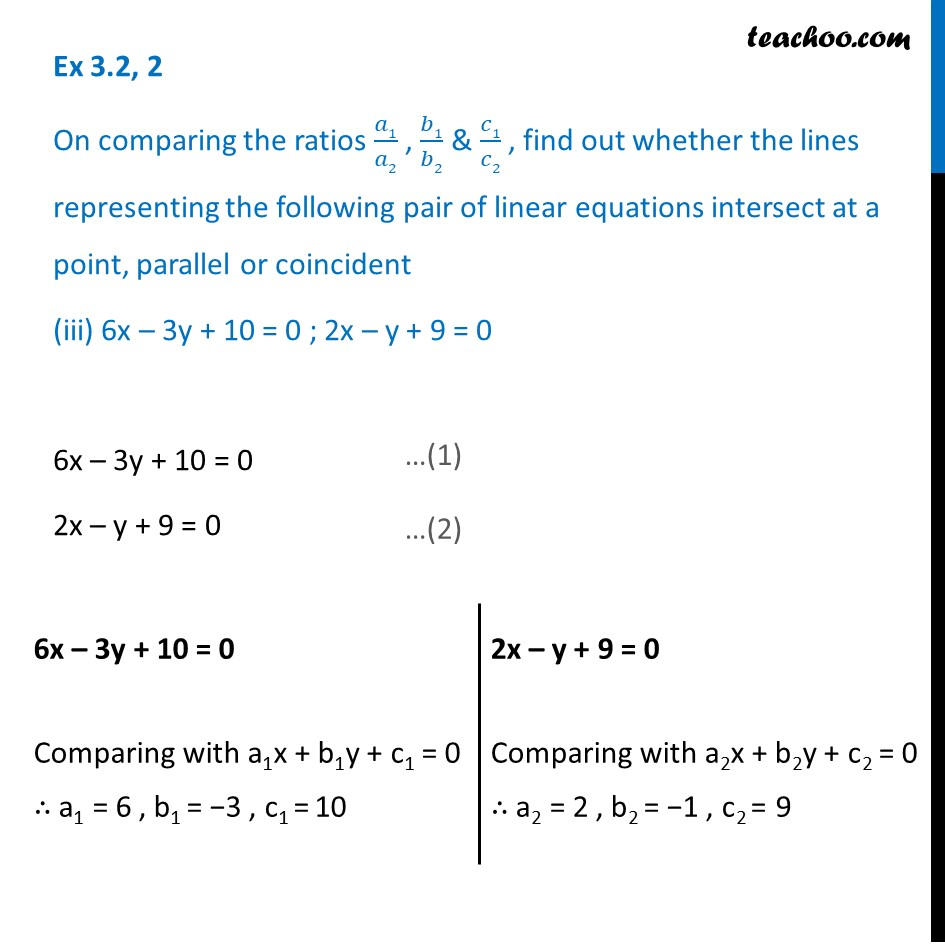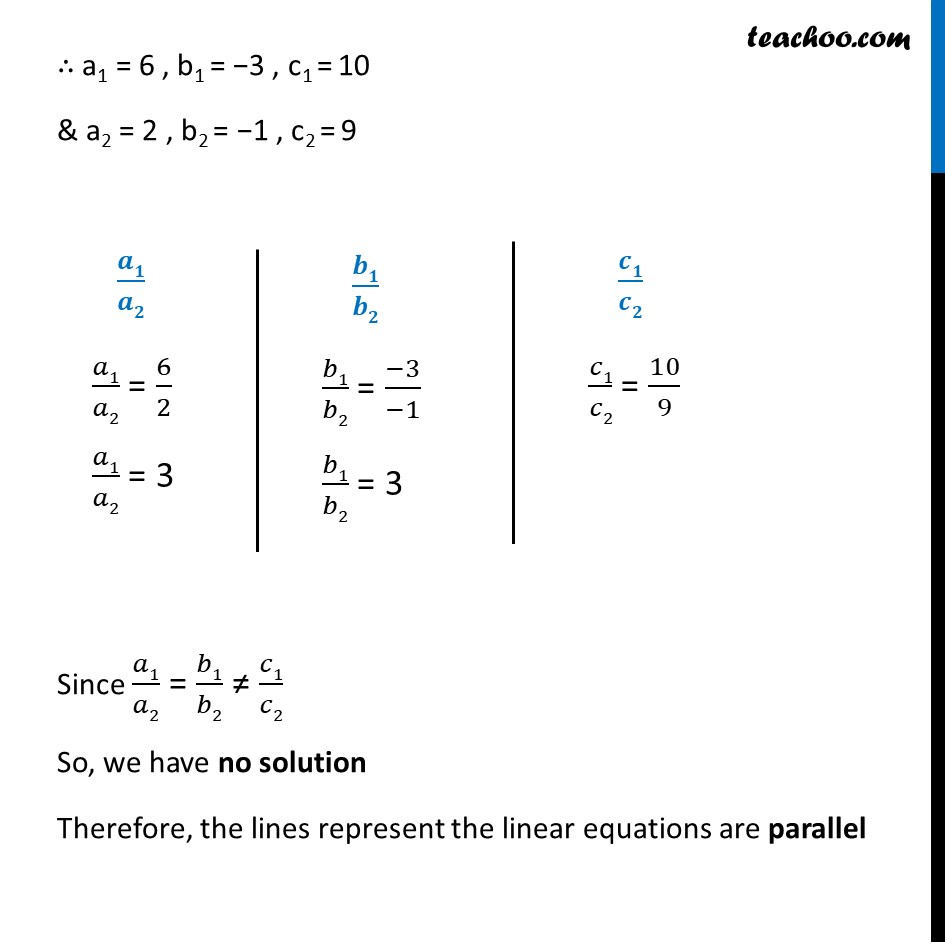1. Chapter 3 Class 10 Pair of Linear Equations in Two Variables (Term 1)
2. Serial order wise
3. Ex 3.2

Transcript

Ex 3.2, 2 On comparing the ratios 𝑎1/𝑎2 , 𝑏1/𝑏2 & 𝑐1/𝑐2 , find out whether the lines representing the following pair of linear equations intersect at a point, parallel or coincident (iii) 6x – 3y + 10 = 0 ; 2x – y + 9 = 0 6x – 3y + 10 = 0 2x – y + 9 = 0 6x – 3y + 10 = 0 Comparing with a1x + b1y + c1 = 0 ∴ a1 = 6 , b1 = −3 , c1 = 10 2x – y + 9 = 0 Comparing with a2x + b2y + c2 = 0 ∴ a2 = 2 , b2 = −1 , c2 = 9 ∴ a1 = 6 , b1 = −3 , c1 = 10 & a2 = 2 , b2 = −1 , c2 = 9 𝒂𝟏/𝒂𝟐 𝑎1/𝑎2 = 6/2 𝑎1/𝑎2 = 3 𝒃𝟏/𝒃𝟐 𝑏1/𝑏2 = (−3)/(−1) 𝑏1/𝑏2 = 3 𝒄𝟏/𝒄𝟐 𝑐1/𝑐2 = 10/9 Since 𝑎1/𝑎2 = 𝑏1/𝑏2 ≠ 𝑐1/𝑐2 So, we have no solution Therefore, the lines represent the linear equations are parallel